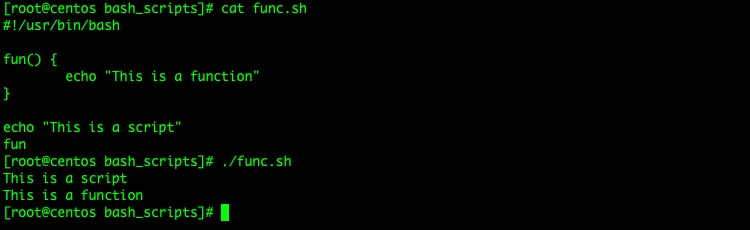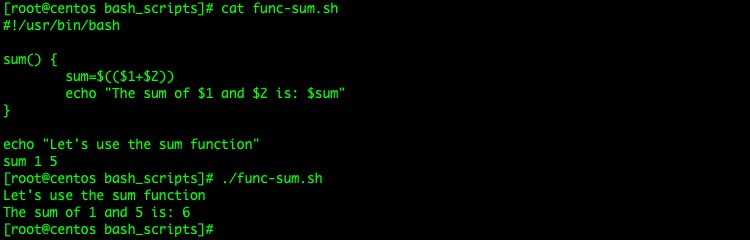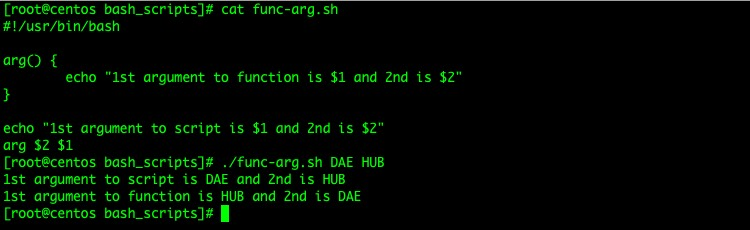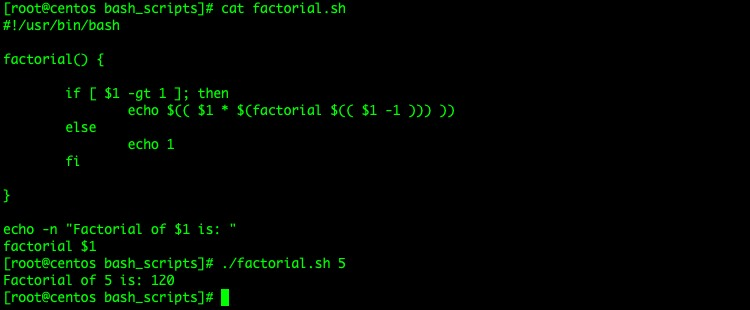Bash 使用函数

```function_name() {
commands
}```

```function_name() {
commands
}

some_other_commands

#FUNCTION CALL
function_name argument;```

```#!/usr/bin/bash

fun() {
echo "This is a function"
}

echo "This is a script"
fun````function_name argument;`

```#!/usr/bin/bash

sum() {
sum=\$((\$1+\$2))
echo "The sum of \$1 and \$2 is: \$sum"
}

echo "Let's use the sum function"
sum 1 5``````#!/usr/bin/bash

arg() {
echo "1st argument to function is \$1 and 2nd is \$2"
}

echo "1st argument to script is \$1 and 2nd is \$2"
arg \$2 \$1```Bash 函数递归调用

```#!/usr/bin/bash

factorial() {

if [ \$1 -gt 1 ]; then
echo \$(( \$1 * \$(factorial \$(( \$1 -1 ))) ))
else
echo 1
fi

}

echo -n "Factorial of \$1 is: "
factorial \$1```